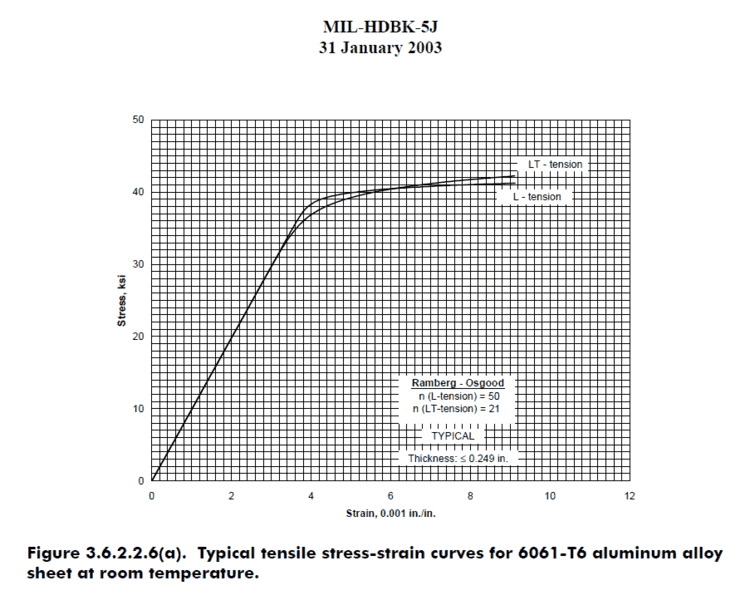# Young's Modulus of Al

My Aim is to determine the youngs modulus of aluminium from the data we get from tensile strength test.
The attached excel sheet contains all the data i am using such as elongation,load value(which is in kN i have converted to N for calculating Stress)
For stress,
I am using the formula
Stress(ε)=Force/Area
For strain,
I am using the formula
Strain(σ)=ΔL/L
My issue's are,
1. The linear portion of the stress-strain curve(engineering) is a vertical line when i plot it with excel.This does not let me calculate the slope.
2. When i have attempted to calculate Youngs modulus with the formula ε/σ,I am getting a value of
758681.8 N/mm2 which is approximately 10x the value which is given online.
My question is whether the values have potentially increased due to various factors/defects or am i making a simple error in calculating stress and strain?

P.S:This is my first post in the forums so do forgive me for making errors/not following protocol.If I did make such errors please tell me how to fix them :)

#### Attachments

• Aluminium.xlsx
1.2 MB · Views: 422

Nidum
Gold Member
I can't open the attachment . Please upload file again in a different format or just paste relevant sections .

I can't open the attachment . Please upload file again in a different format or just paste relevant sections .
I can't paste relevant section since the excel sheet has 2400 or so rows for the values of load and elongation.(unless its possible to do so?)
Can you suggest a different file type?

Nidum
Gold Member
One of the ODF formats like .ods would probably be ok if available .

If not mentioned specifically see if there is an 'Open Office' format option .

Site says OpenDocument Spreadsheet format is not permitted.
The attached file type is'Strict OPEN XML spreadsheet'.

#### Attachments

• Aluminium2.xlsx
156.1 KB · Views: 243
Nidum
Gold Member
The latest version does not open either but no matter as I've now found some software that was able open the original file .

Please explain exactly what you did in this experiment , why there are so many duplicated test results yet so few actual changes of load and measured extension and what the units are for the values in the tables ?

The latest version does not open either but no matter as I've now found some software that was able open the original file .

Please explain exactly what you did in this experiment , why there are so many duplicated test results yet so few actual changes of load and measured extension and what the units are for the values in the tables ?
The test was pretty straight forward,I planted the specimen on the Universal Testing Machine for tensile strength testing.
The 'duplicated' values of load and elongation are the values the the universal tester recorded during the test from start till point of rupture.
The initial units are in kN and in mm,
I've converted kN to N for calculating stress(x1000) divided by area.

Mech_Engineer